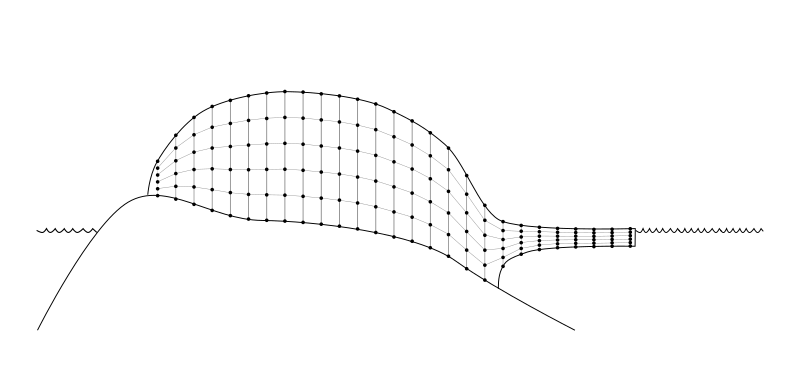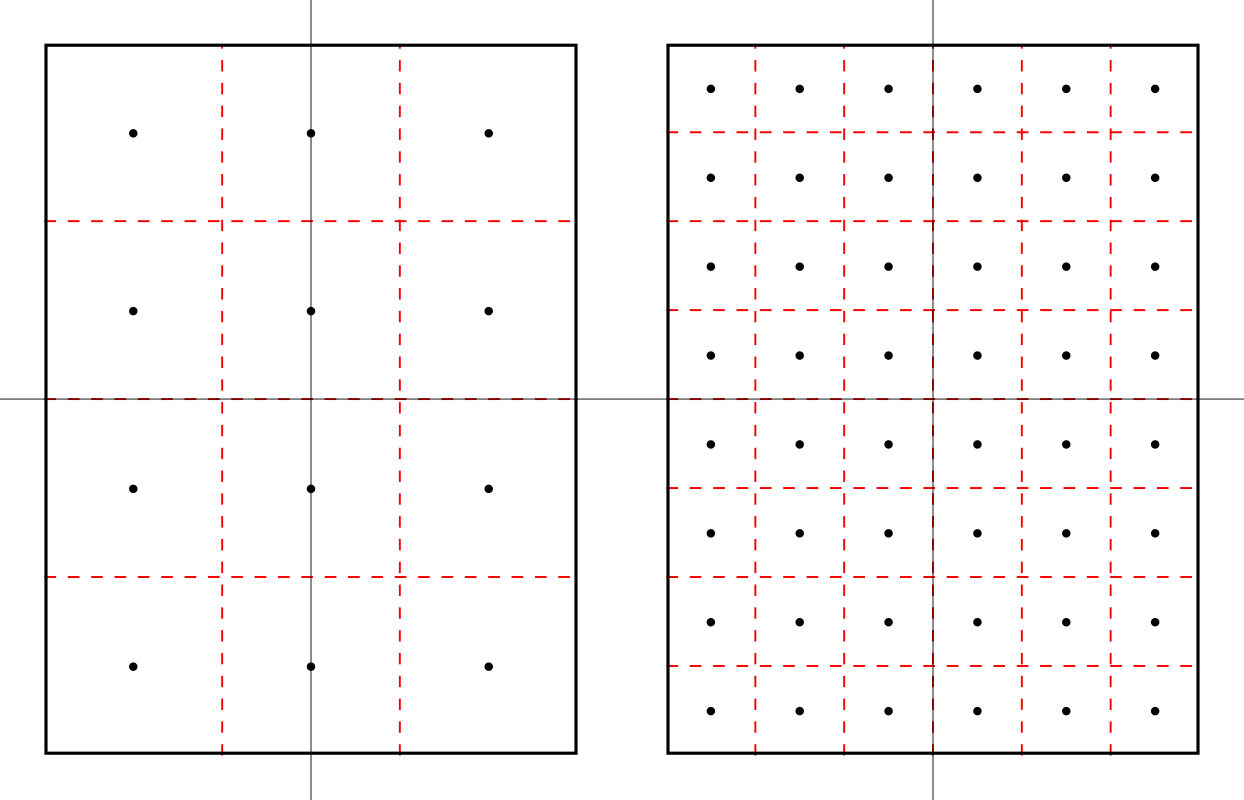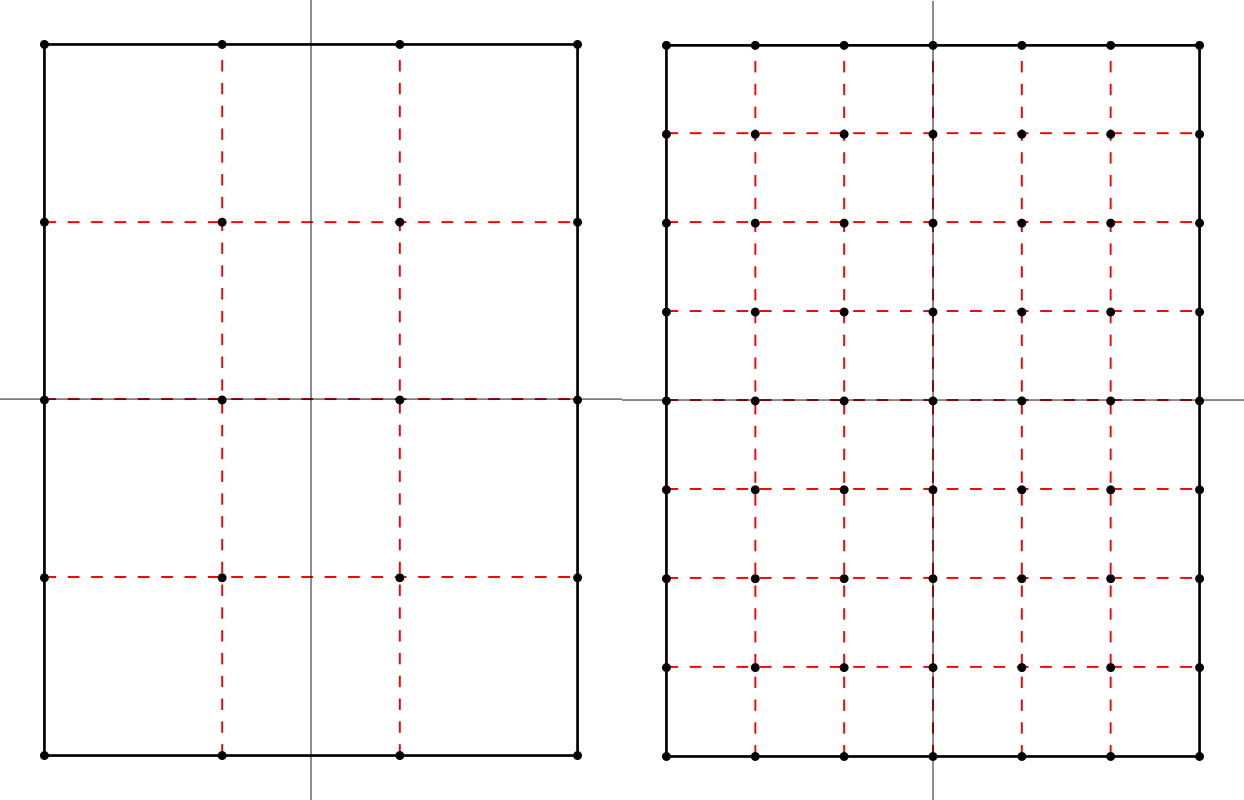# Spatial grid¶

The PISM grid covering the computational box is equally spaced in horizontal ($$x$$ and $$y$$) directions. Vertical spacing in the ice is quadratic by default but optionally equal spacing can be chosen; set using grid­.ice_vertical_spacing at bootstrapping. The bedrock thermal layer model always uses equal vertical spacing.

The grid is described by four numbers, namely the number of grid points grid­.Mx in the $$x$$ direction, the number grid­.My in the $$y$$ direction, the number grid­.Mz in the $$z$$ direction within the ice, and the number grid­.Mbz in the $$z$$ direction within the bedrock thermal layer. These are specified by options -Mx, -My, -Mz, and -Mbz, respectively. Note that Mx, My, Mz, and Mbz all indicate the number of grid points so the number of grid spaces are one less.

The lowest grid point in a grounded column of ice, at $$z=0$$, coincides with the highest grid point in the bedrock, so grid­.Mbz must always be at least one.Fig. 18 PISM’s vertical grid with uniform $$z$$ spacing. See On the vertical coordinate in PISM, and a critical change of variable for details.

Some PISM components (currently: the Blatter stress balance solver) use a geometry-following vertical grid with uniform vertical spacing withing each column. See Fig. 19.Fig. 19 The “sigma” vertical grid used by PISM’s Blatter solver

Choosing Mbz $$>1$$ is required to use the bedrock thermal model. When a thermal bedrock layer is used, the distance Lbz is controlled by the -Lbz option. Note that grid­.Mbz is unrelated to the bed deformation model (glacial isostasy model); see section Earth deformation models.

In the quadratically-spaced case the vertical spacing near the ice/bedrock interface is about four times finer than it would be with equal spacing for the same value of Mz, while the spacing near the top of the computational box is correspondingly coarser. For a detailed description of the spacing of the grid, see the documentation on IceGrid::compute_vertical_levels() in the PISM class browser.

The user should specify the grid when using -bootstrap or when initializing a verification test (section Verification) or a simplified-geometry experiment (section Simplified geometry experiments). If one initializes PISM from a saved model state using -i then the input file determines all grid parameters. For instance, the command

pismr -i foo.nc -y 100


should work fine if foo.nc is a PISM output file. Because -i input files take precedence over options,

pismr -i foo.nc -Mz 201 -y 100


will give a warning that “PISM WARNING: ignoring command-line option '-Mz'”.

## Grid registration¶

PISM’s horizontal computational grid is uniform and (by default) cell-centered.1

This is not the only possible interpretation, but it is consistent with the finite-volume handling of mass (ice thickness) evolution is PISM.

Consider a grid with minimum and maximum $$x$$ coordinates $$x_\text{min}$$ and $$x_\text{max}$$ and the spacing $$\dx$$. The cell-centered interpretation implies that the domain extends past $$x_\text{min}$$ and $$x_\text{max}$$ by one half of the grid spacing, see Fig. 20.Fig. 20 Computational grids using the default (center) grid registration.

Left: a coarse grid. Right: a finer grid covering the same domain.

The solid black line represents the domain boundary, dashed red lines are cell boundaries, black circles represent grid points.

When getting the size of the domain from an input file, PISM will compute grid parameters as follows:

\begin{align}\begin{aligned}\dx &= x_1 - x_0\\L_x &= \frac12 ((x_\text{max} - x_\text{min}) + \dx).\end{aligned}\end{align}

This is not an issue when re-starting from a PISM output file but can cause confusion when specifying grid parameters at bootstrapping and reading in fields using “regridding.”

For example:

> pismr -eisII A -grid.registration center \
-Lx 10 -Mx 4 \
-y 0 -verbose 1 \
-o grid-test.nc
> ncdump -v x grid-test.nc | tail -2 | head -1
x = -7500, -2500, 2500, 7500 ;


Note that we specified the domain half width of 10 km and selected 4 grid points in the $$x$$ direction. The resulting $$x$$ coordinates range from $$-7500$$ meters to $$7500$$ meters with the grid spacing of $$5$$ km.

In summary, with the default (center) grid registration

(2)\begin{align}\begin{aligned}\dx &= \frac{2 L_x}{M_x},\\x_\text{min} &= x_c - L_x + \frac12 \dx,\\x_\text{max} &= x_c + L_x - \frac12 \dx,\end{aligned}\end{align}

where $$x_c$$ is the $$x$$-coordinate of the domain center.

Note

One advantage of this approach is that it is easy to build a set of grids covering a given region such that grid cells nest within each other as in Fig. 20. In particular, this makes it easier to create a set of surface mass balance fields for the domain that use different resolutions but have the same total SMB.

Compare this to

> pismr -eisII A -grid.registration corner \
-Lx 10 -Mx 5 \
-y 0 -verbose 1 \
-o grid-test.nc
> ncdump -v x grid-test.nc | tail -2 | head -1
x = -10000, -5000, 0, 5000, 10000 ;


Here the grid spacing is also 5 km, although there are 5 grid points in the $$x$$ direction and $$x$$ coordinates range from $$-10000$$ to $$10000$$.

With the “corner” grid registration

(3)\begin{align}\begin{aligned}\dx &= \frac{2 L_x}{M_x - 1},\\x_\text{min} &= x_c - L_x,\\x_\text{max} &= x_c + L_x.\end{aligned}\end{align}

See Fig. 21 for an illustration.Fig. 21 Computational grids using the corner grid registration.

Left: a coarse grid. Right: a finer grid covering the same domain.

To switch between (2) and (3), set the configuration parameter grid­.registration.

## Grid projections¶

PISM can use the PROJ library (see Required tools and libraries) and projection information to compute

• latitudes and longitudes of grid points (variables lat and lon), and

• latitudes and longitudes of cell corners (variables lat_bnds and lon_bnds).

To use this feature, compile PISM with PROJ and add the global attribute proj containing the parameter string describing the projection to the input file.

For example, the input file pism_Greenland_5km_v1.1.nc in Getting started: a Greenland ice sheet example has the following:

> ncdump -h pism_Greenland_5km_v1.1.nc | grep :proj
:proj = "+proj=stere +lat_0=90 +lat_ts=71 +lon_0=-39 +k=1 +x_0=0 +y_0=0 +ellps=WGS84 +towgs84=0,0,0,0,0,0,0 +units=m +no_defs" ;


The spinup run in that example disables the code re-computing longitude, latitude grid coordinates using projection information to avoid the dependency on PROJ (look for -grid.recompute_longitude_and_latitude in the command). If we remove this option, PISM will report the following.

> pismr -i pism_Greenland_5km_v1.1.nc \
-bootstrap -Mx 76 -My 141 -Mz 101 -Mbz 11 ... \
-grid.recompute_longitude_and_latitude true ... -o output.nc
...
* Got projection parameters "+proj=stere +lat_0=90 +lat_ts=71 +lon_0=-39 +k=1 +x_0=0 +y_0=0 +ellps=WGS84 +towgs84=0,0,0,0,0,0,0 +units=m +no_defs" from "pism_Greenland_5km_v1.1.nc".
* Computing longitude and latitude using projection parameters...
...
... done with run
Writing model state to file output.nc'...


If the proj attribute contains the string “+init=epsg:XXXX” where XXXX is 3413, 3031, or 26710, PISM will also create a CF-conforming mapping variable describing the projection in use.

“Mapping” variables following CF metadata conventions in input files are copied to output files (including -extra_files) but are not used to compute latitude/longitude coordinates.

To simplify post-processing and analysis with CDO PISM adds the PROJ string (if known) to the mapping variable, putting it in the proj_params attribute.

> ncdump -h g20km_10ka_hy.nc | grep mapping:

mapping:ellipsoid = "WGS84" ;
mapping:grid_mapping_name = "polar_stereographic" ;
mapping:false_easting = 0. ;
mapping:false_northing = 0. ;
mapping:latitude_of_projection_origin = 90. ;
mapping:standard_parallel = 71. ;
mapping:straight_vertical_longitude_from_pole = -39. ;
mapping:proj_params = "+proj=stere +lat_0=90 +lat_ts=71 +lon_0=-39 +k=1 +x_0=0 +y_0=0 +ellps=WGS84 +towgs84=0,0,0,0,0,0,0 +units=m +no_defs" ;


## Parallel domain distribution¶

When running PISM in parallel with mpiexec -n N, the horizontal grid is distributed across $$N$$ processes 2. PISM divides the grid into $$N_x$$ parts in the $$x$$ direction and $$N_y$$ parts in the $$y$$ direction. By default this is done automatically, with the goal that $$N_x\times N_y = N$$ and $$N_x$$ is as close to $$N_y$$ as possible. Note that $$N$$ should, therefore, be a composite (not prime) number.

Users seeking to override this default can specify $$N_x$$ and $$N_y$$ using the -Nx and -Ny command-line options.

Once $$N_x$$ and $$N_y$$ are computed, PISM computes sizes of sub-domains $$M_{x,i}$$ so that $$\sum_{i=1}^{N_x}M_{x,i} = M_x$$ and $$M_{x,i} - \left\lfloor M_x / N_x \right\rfloor < 1$$. To specify strip widths $$M_{x,i}$$ and $$M_{y,i}$$, use command-line options -procs_x and -procs_y. Each option takes a comma-separated list of numbers as its argument. For example,

mpiexec -n 3 pismr -eisII A -Mx 101 -My 101 \
-Nx 1 -procs_x 101 \
-Ny 3 -procs_y 20,61,20


splits a $$101 \times 101$$ grid into 3 strips along the $$x$$ axis.

To see the parallel domain decomposition from a completed run, see the rank variable in the output file, e.g. using -o_size big. The same rank` variable is available as a spatial diagnostic field (section Spatially-varying diagnostic quantities).

Footnotes

1

This is consistent with the CF Conventions document for data-sets without cell bounds: “If bounds are not provided, an application might reasonably assume the gridpoints to be at the centers of the cells, but we do not require that in this standard.”

2

In most cases one process corresponds to one “core” of your computer.

 Previous Up Next